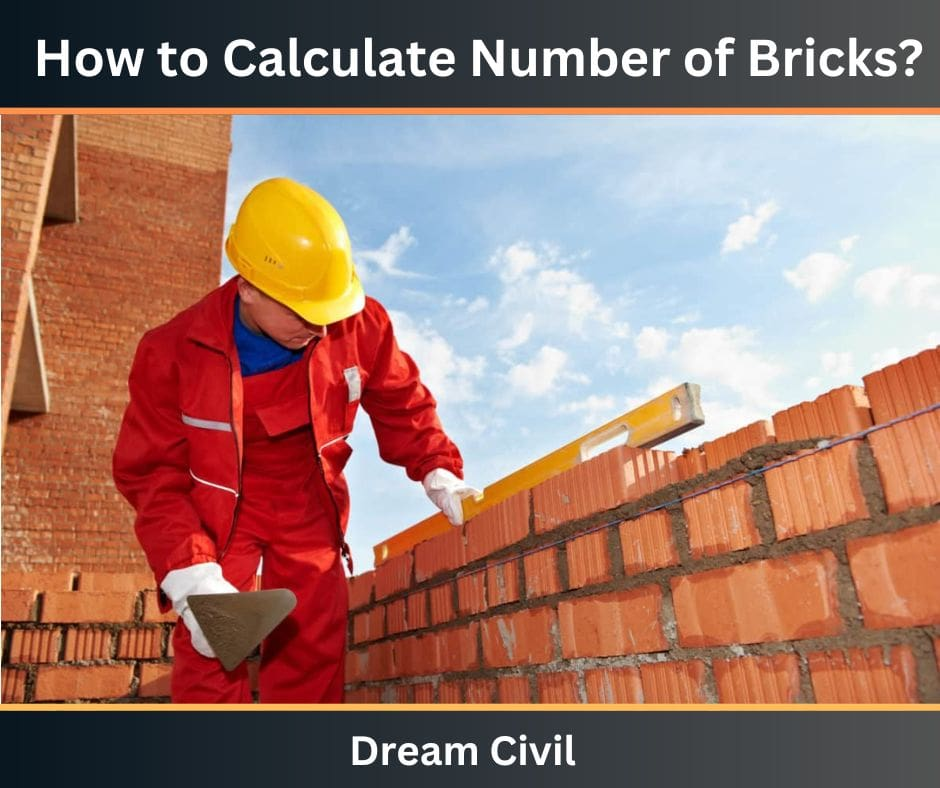Tuesday, October 3, 2023

# How to Calculate Number of Bricks : Single and Double Layer Bricks Layer | Convert sq.m to sq.ft

Brick is a construction material that is used to build walls, pavement, and other elements in the construction field. Bricks are artificial materials made with the help of dried clay, sand, lime, and other ingredients.

It is mainly used to build walls and boundaries walls.

According to the Indian Standard size, its dimensions are (19 cm x 9 cm x 9 cm) of the brick when laid flat. In the US generally, Modular bricks are used whose standard size is 194 × 92 × 57 mm. And, the standard size of brick in the UK is 215 mm x 102.5 mm x 65 mm.

The surface on which mortar is placed on both sides is known as a Bed of brick.

### 1. Types of Brick Masonry

There are two types of brick wall masonry;

1. Singular or half-brick wall

The half or singular brick wall is prepared of a single bricklayer, it has a thickness of about 100 mm or 4″ also known as a 4″ brick masonry wall.

2. Double or full brick wall

The full or double brick wall is prepared of two bricklayers, it has a thickness of about 200mm or 8″ also known as 8″ brick masonry wall.### 2. How to Calculate Number of Bricks in a Single Layer Brick Wall

The Brick calculation in the meter for a single layer or half 4″ brick wall/ calculate the number of bricks required for the wall in the following ways:-

a. Measure the length and height of the brick wall in meters, Consider this would be 5 m in length by 4 m in high.

b. Calculate the square meter of the wall by multiplying the length and height of the wall to get a square meter such as 5 m × 4 m = 20 m2.

c.You need to multiply the resulting square meter by 50 to figure out the number of modular bricks needed for a single layer or half brick wall such as 20 ×50 = 1000 nos bricks, thus you needed 1000 nos modular bricks for 5 m × 4 m single layer or half 4″ brick wall.

### 3. How to Calculate the Number of Bricks in a Double Layer Brick   Wall

How to calculate brick in a double layer brick wall in meter brick calculation in the meter for a double layer or full 9″ brick wall/ calculate the number of bricks needed for the wall in the following ways:-

a. Measure the length and height of the brick wall in meters, assume this would be 5 m in length by 4 m in high

b. calculate the square meter of the wall by multiplying the length and height of the wall to get a square meter such as 5 m × 4 m = 20 m2.

c. You need to multiply the resulting square meter by 100 to figure out the number of modular bricks required for a double layer or full 9″ brick wall such as 20 ×100 = 2000 nos bricks, thus you required 2000 nos modular bricks for 5 m×4 m double layer or full 9″ brick wall.

This was for the How to Calculate Number of Bricks?

### 4. Convert m2 to ft2

Multiply the area value by 10.764 to change it into square feet.

### 5. References

1. Content Filter & Authenticity Checking Team, Dream Civil International

(Our team checks every content & detail to maintain quality.)

 Read Also: Water Required For M20 ConcreteHe is a founder and lead author of Dream Civil International and his civil engineering research articles has been taken as source by world's top news and educational sites like USA Today, Time, The richest, Wikipedia, etc.
Latest Articles

Related Articles